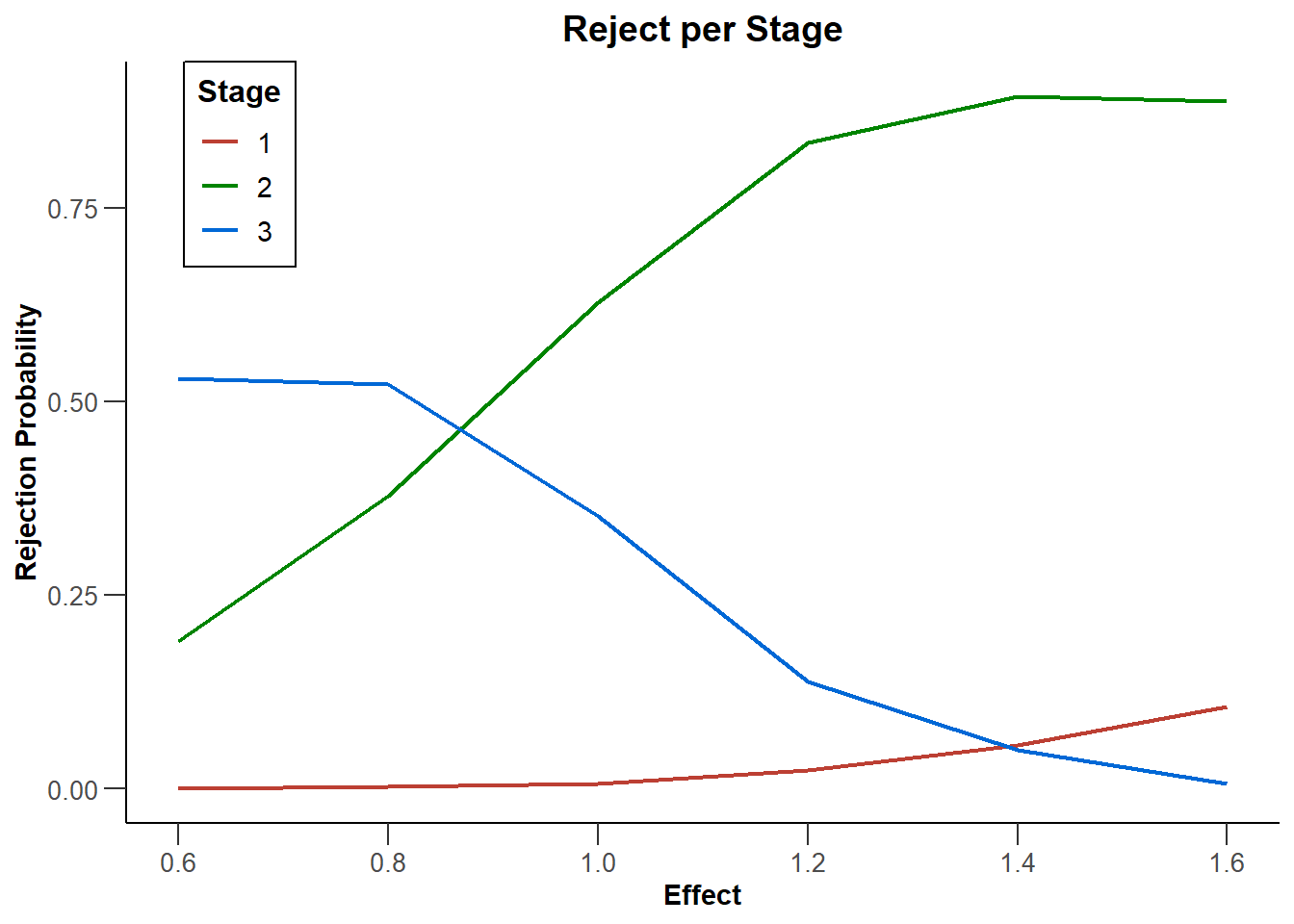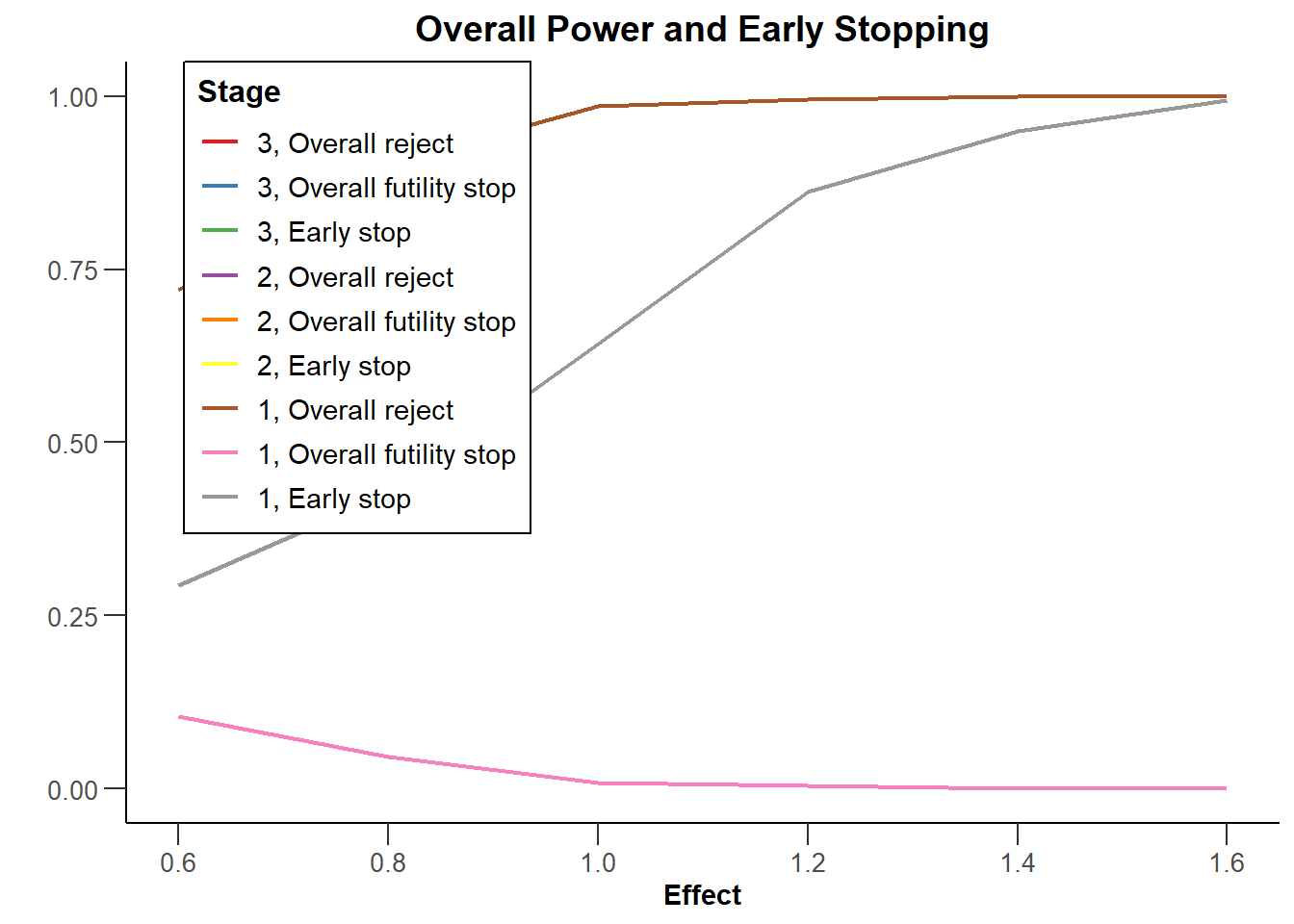Free examples and use-cases:   rpact vignettes

# Summary

This R Markdown document provides many different examples for creating one- and multi-arm simulation result plots with rpact and ggplot2.

# 1 Preparation

``````library(rpact)
packageVersion("rpact") ``````
``##  '3.2.1'``

# 2 Simulation results base

## 2.1 Simulation results base - means

``````maxNumberOfSubjects <- 90
informationRates <- c(0.2, 0.5, 1)
plannedSubjects <- round(informationRates * maxNumberOfSubjects)
design <- getDesignInverseNormal(futilityBounds = c(-0.5, 0.5), informationRates = informationRates)
x <- getSimulationMeans(design = design, groups = 2, meanRatio = TRUE, thetaH0 = 0.4,
plannedSubjects = plannedSubjects, maxNumberOfIterations = 500,
allocationRatioPlanned = 3, stDev = 1.5, seed = 1234567890)
plot(x, type = "all", grid = 0)``````